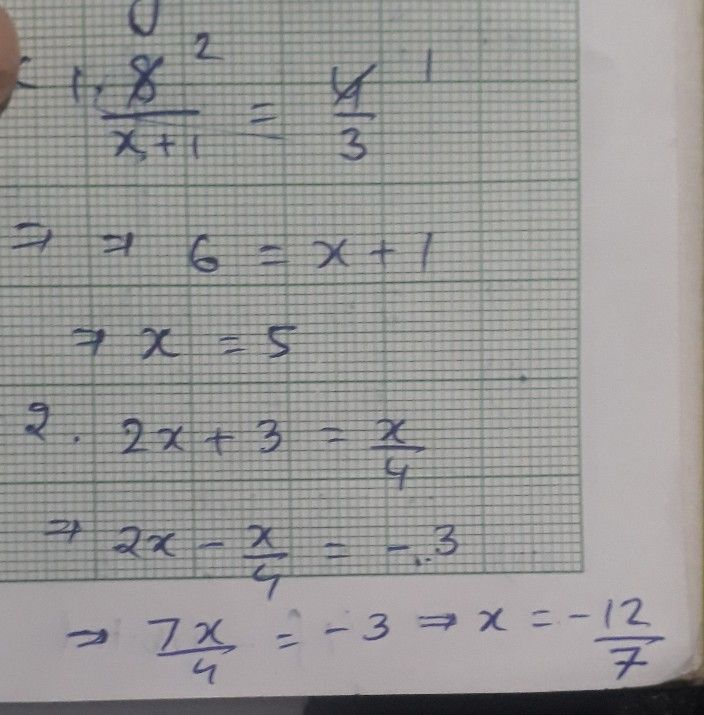Symbol
ProblemPractice $Norkshcet$ Solving Rational Equations and Inequalities Solve each equation. Check extraneous solutions for rational equations. Write $y01\pi$ answer $in$ interval notation for rational inequalities. LEVEL 1 1. 2. $\dfrac {8} {x+1}=\dfrac {4} {3}$ $2x+3=\dfrac {x} {4}$ 3. 4. $\dfrac {x-4} {x+5}\leq 0$ $\dfrac {x+3} {3x-6}>0$ -
10th-13th grade
Calculus
Search count: 143
SolutionQanda teacher - Sid711Student
where's the number 3 and 4 sir?Qanda teacher - Sid711
pls don't ask other questions as I may be banned for answering multiple questionsStudent
okay sir, thank u